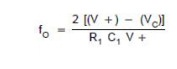Home | | Linear Integrated Circuits | Voltage Controlled Oscillator

# Voltage Controlled Oscillator

If the modulating voltage at pin 5 is increased, the voltage at pin 6 also increases, resulting in less voltage across R1 and thereby decreasing the charging current.

Voltage Controlled Oscillator:Referring to the circuit in the above figure, the capacitor c1 is linearly charged or discharged by a constant current source/sink. The amount of current can be controlled by changing the voltage vc applied at the modulating input (pin 5) or by changing the timing resistor R1 external to the IC chip. The voltage at pin 6 is held at the same voltage as pin 5.

Thus, if the modulating voltage at pin 5 is increased, the voltage at pin 6 also increases, resulting in less voltage across R1 and thereby decreasing the charging current.

The voltage across the capacitor C1 is applied to the inverting input terminal of Schmitt trigger via buffer amplifier. The output voltage swing of the Schmitt trigger is designed to Vcc and 1.5 Vcc. If Ra = Rb in the positive feedback loop, the voltage at the non-inverting input terminal of Schmitt trigger swings from 0.5 Vcc to 0.25 Vcc.

When the voltage on the capacitor c1 exceeds 0.5 Vcc during charging, the output of the Schmitt trigger goes LOW (0.5 Vcc). The capacitor now discharges and when it is at 0.25 Vcc, the output of Schmitt trigger goes HIGH (Vcc). Since the source and sink currents are equal, capacitor charges and discharges for the same amount of time. This gives a triangular voltage waveform across c1 which is also available at pin 4.

The square wave output of the Schmitt trigger is inverted by buffer amplifier at pin 3. The output waveforms are shown near the pins 4 and 3.

The output frequency of the VCO can be given as follows:where V+ is Vcc.

The output frequency of the VCO can be changed either by (i) R1, (ii) c1 or (iii) the voltage vc at the modulating input terminal pin 5. The voltage vc can be varied by connecting a R1R2 circuit as shown in the figure below. The components R1and c1 are first selected so that VCO output frequency lies in the centre of the operating frequency range.

Now the modulating input voltage is usually varied from 0.75 Vcc to Vcc which can produce a frequency variation of about 10 to 1.

The signetics NE/SE 560 series is monolithic phase locked loops. The SE/NE 560, 561, 562, 564, 565 & 567 differ mainly in operating frequency range, poser supply requirements & frequency & bandwidth adjustment ranges.

Tags : Phase Locked Loop (PLL) , Linear Integrated Circuits : Analog Multiplier and PLL
Study Material, Lecturing Notes, Assignment, Reference, Wiki description explanation, brief detail
Linear Integrated Circuits : Analog Multiplier and PLL : Voltage Controlled Oscillator | Phase Locked Loop (PLL)